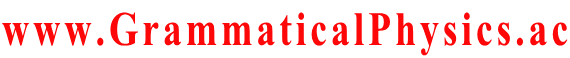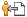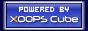Welcome SourceCodeOf_HumanGenome
www.GrammaticalPhysics.ac
Account
Access Detector
1 user(s) are online (1 user(s) are browsing Forum)

Members: 1
Guests: 0

SourceCodeOf_HumanGenome more...

# Theory of Quantum History Entangled in Time-like Direction

SourceCodeOf_HumanGenome > Line Integral in Function Space @ 2013/5/26 16:32
ξ(□, x) represents a function which is a point in the function space for each x.

So, ξ is a parametric representation of a line in the function space, and x is a parameter of it.

By thinking of t as an index of a coordinate in the function space, η(x) = √{α∫dt [∂ξ(t,x)/∂x]2}
is understood as ds/dx where ds is the length of a line element.

η(x) is chosen to be 1 in the definition of the probability formula.

[dar(f; a, a + 1/α)]pre Φ[χ]
= ∫dx η(x) f(x)* Φ[ξ(□,x)]
= ∫dx f(x)* Φ[ξ(□,x)]

---
Last edited at 2013/05/31/17:34JST
SourceCodeOf_HumanGenome > Twist Remove Normalization @ 2013/5/27 11:23
I suppose that a general definition of the twist remove normalization for a functional Φ is given by the condition:
∫Dχ Φ[χ]* Φ[χ] = 1,
(d/dε) ∫Dχ Φ[χ]* Φ[χ(□ - ε)] |ε = 0 = 0,
where χ(□ - ε) is a function such that
[χ(□ - ε)](t) = χ(t - ε) for all t.

Possibly, a general definition of the twist remove normalization for a functional Φ is given by the condition:
∫Dχ Φ[χ]* Φ[χ] = 1,
limε → 0 ∫Dχ Φ[χ]* Φ[χ(□ - ε)] = 1
because the phase factor exp[-⊿t(i/h)＜j|H]|j＞] in Problems in Grammatical Physics > Quantum Field Theory on the Time-Axis > Quadratic Formula corresponds not to (d/dε) ∫Dχ Φ[χ]* Φ[χ(□ - ε)] |ε = 0 but to limε → 0 ∫Dχ Φ[χ]* Φ[χ(□ - ε)].

Possibly, the twist remove normalization may not be necessary for Φ in the case that we use exp∫dt instead of Πt.
---
Last edited at 2013/05/28/16:05JST
SourceCodeOf_HumanGenome > Examine Probability Formula @ 2013/5/28 16:29
Φ[χ] = Σj exp[α∫dt φj(χ(t), t)],

[dar(f; a, a + 1/α)]pre Φ[χ]
= ∫dx f(x)* Φ[ξ(□, x)]
= ∫dx f(x)* Σj exp[α∫dt φj(ξ(t, x), t)]
= Σj ∫dx f(x)* exp[α∫aa+1/α dt φj(x, t)] exp[α∫t'＜a or t'≧a+1/α dt' φj(χ(t'), t')]

dar(f; a, a + 1/α) Φ[χ(a,a+1/α)]
= [dar(f; a, a + 1/α)]pre Φ[χ]
= Σj ∫dx f(x)* exp[α∫aa+1/α dt φj(x, t)] exp[α∫t'＜a or t'≧a+1/α dt' φj(χ(t'), t')]
= Σj ∫dx f(x)* exp[α∫aa+1/α dt φj(x, t)] exp[α∫-∞a dt' φj(a,a+1/α)(t'), t') + α∫a dt' φj(a,a+1/α)(t'), t'+1/α)]
≒ Σj ∫dx f(x)* exp φj(x, a)
exp[ Σk＜0 φj(a,a+1/α)(a + k/α), a + k/α) + Σk≧0 φj(a,a+1/α)(a + k/α), a + (k + 1)/α)] ∵ α ≫ 1

dar(f; a, a + 1/α) Φ[χ]
≒ Σj ∫dx f(x)* exp φj(x, a) exp[ Σk＜0 φj(χ(a + k/α), a + k/α) + Σk≧0 φj(χ(a + k/α), a + (k + 1)/α)]

Let exp φj be a solution of the old Schrodinger equation for each j.
Let {exp φ1(□, t), exp φ2(□, t), ・・・} be an orthonormal basis of the state space of the old quantum mechanics for each t.

∫Dχ dar(g; b, b + 1/α) Φ[χ]・{dar(f; a, a + 1/α) Φ[χ]}*
≒ Σj,j'k∫dχ(a+k/α)]
∫dx' g(x')* exp φj'(x', b) exp[ Σk'＜0 φj'(χ(b + k'/α), b + k'/α) + Σk'≧0 φj'(χ(b + k'/α), b + (k' + 1)/α)]
{∫dx f(x)* exp φj(x, a) exp[ Σk＜0 φj(χ(a + k/α), a + k/α) + Σk≧0 φj(χ(a + k/α), a + (k + 1)/α)]}*
= Σj,j' ∫dx' g(x')* exp φj'(x', b) ∫dx f(x) [exp φj(x, a)]*
Πk＜0 ∫dχ(a+k/α) [exp φj(χ(a + k/α), a + k/α)]* [exp φj(χ(a + k/α), a + k/α)]
Π0≦k＜α(b-a) ∫dχ(a+k/α) [exp φj(χ(a + k/α), a + (k + 1)/α)]* [exp φj(χ(a + k/α), a + k/α)]
Πk≧α(b-a) ∫dχ(a+k/α) [exp φj(χ(a + k/α), a + k/α)]* [exp φj(χ(a + k/α), a + k/α)]
= Σj,j' ∫dx' g(x')* exp φj'(x', b) ∫dx f(x) [exp φj(x, a)]*
(δj,j') Π0≦k＜α(b-a) ∫dχ(a+k/α) [exp φj(χ(a + k/α), a + (k + 1)/α)]* [exp φj(χ(a + k/α), a + k/α)]
= Σj ＜g|j,b＞＜j,a|f＞ Π0≦k＜α(b-a) ＜j,a+(k+1)/α|j,a+k/α＞
≒ Σj ＜g|j,b＞＜j,a|f＞ ∵ ※
= Σj ＜g|U(b,a)|j,a＞＜j,a|f＞
= ＜g|U(b,a)|f＞
if α(b - a) ∈ N.

---※---
Π0≦k＜α(b-a) ∫dχ(a+k/α) [exp φj(χ(a + k/α), a + (k + 1)/α)]* [exp φj(χ(a + k/α), a + k/α)]
= Π0≦k＜α(b-a) ＜j,a+(k+1)/α|j,a+k/α＞
≒ Π0≦k＜α(b-a) ＜j,a+k/α|j,a+k/α＞ ∵the twist remove normalization
= ＜j,a|j,a＞α(b-a)
= 1
---

However, notice that the new grammar version of the Schrödinger equation has no solution such that Φ[χ] = Σj exp[α∫dt φj(χ(t), t)].

A few approximations caused by the finiteness of α shift the result from the one in the old quantum mechanics.
Such an approximation is not needed if α is infinity.
I don't think that it is a prediction of the new theory distinct from the old quantum mechanics.
I think that it should be understood as the fact that a quantum history and a measurement are less related to each other than in the old quantum mechanics.

A prediction of the new theory distinct from the old quantum mechanics will be caused by the entanglement of a quantum history in a time-like direction.

---
Last edited at 2013/06/03/16:48JST

Category
Forum
Global

Notification method is private message [Change]

Site Search
Site Info
WebmasterswooderRecommend Us